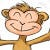# Interpolation with Generative Models

Source: Deep Learning on Medium

## How generative models learn to create something new

In this post I am going to write about generative models. It’s gonna cover the dichotomy between generative and discriminative models, and how generative models can really learn the essence of objects of interest by being able to perform interpolations.

### 0. Generative models (G) versus discriminative models (D)

To be honest, I merely started to contemplate about the nature of statistical and Machine Learning models after the Generative Adversarial Nets (GANs) took off. In the original version of GAN, let’s term it vanilla GAN, you have a generative network (G) that is generating synthetic data from Gaussian noise and a discriminative network (D) that is trying to distinguish the fake from the real. Obviously, G and D in the vanilla GAN are generative and discriminative model, respectively. In fact, GAN is perhaps the first ML algorithm that harmonizes generative and discriminative models, which learns the parameters of both models through the innovative adversarial training.

So much for my own experience, what are generative and discriminative models? Intuitively, generative models are trying to abstract the some generalizable patterns of some collection of objects whereas discriminative models attempt to find the differences between collections. Concretely, in the context of a classification problem, for instance, generative models would learn the characteristics of each classes whereas discriminative models would find the decision boundaries that best separate the classes. More formally, let’s represent an instance as a feature vector x labeled by some scalar value y,generative models learn the joint probability distribution p(x, y), whereas discriminative models learn conditional probability distribution p(y|x).

There are also some interesting generator-discriminator pairs to think about:

• Binary classification: Naive Bayes vs Logistic Regression
• Sequence modeling: Hidden Markov Model vs Conditional Random Fields

It’s also worth mentioning that most traditional ML classifiers are discriminative models, including Logistic Regression, SVM, Decision Trees, Random Forest, LDA. Discriminative models are parsimonious in terms of the parameters to be learned, and has been demonstrated to have superior performance over their generative counterpart in many classification tasks.

But I’d like to argue that learning to tell one class from another is not really learning, as it usually can’t work when situated into another context. For example, a discriminative classifier trained to distinguish cats and birds with exceptional accuracy may fail miserably when an unseen class, dog, is added to the test set, as the discriminative classifier may simple learn that something with four legs are cats and otherwise birds.

To further illustrate what Generative and Discriminative models really learn, let’s consider the simplest classification models from each, Naive Bayes and Logistic Regression. The following figure visualize the learned “knowledge” by Naive Bayes and Logistic Regression classifiers on a binary classification problem.

Naive Bayes classifier learns the mean and variance vectors for the two classes whereas Logistic Regression learns the slope and intercept of a linear boundary that optimally separate the two classes. With the means and variances learned from the Naive Bayes classifier, we can generate synthetic samples for each class by sampling from the multivariate Gaussian distribution. This is similar to generating synthetic samples using GANs, but obviously Naive Bayes won’t be able to generate any high quality high dimensional images because it is too naive to model the features dependently.

### 1. Generative models

I briefly touched on Naive Bayes algorithm, arguably the simplest form of generative model. Modern generative models usually involves deep neural network architectures, hence termed deep generative models. There are three types of deep generative models:

#### 1.1. VAE

VAE was introduced by Kingma & Welling, 2014, as an probabilistic extension of the autoencoder (AE). It has the following three additional features over vanilla AE:

1. Probabilistic encoder qϕ(z|x) and decoder pθ(x|z)
2. A prior probability distribution for the latent space (the bottleneck layer of the AE): pθ(z)
3. A latent loss defined by Kullback-Leibler divergence: D(qϕ(z|x)‖pθ(z|x)) to quantify the distance between these two probability distributions

#### 1.2. GANs

GAN was introduced by Goodfellow et al., 2014 and is composed of a pair of Generator and Discriminator networks playing a minimax game against each other. Many variants of GANs have been developed, such as Bidirectional GAN (BiGAN), CycleGAN, InfoGAN, Wasserstein GAN and the list keeps growing.

BiGAN is particularly attractive in that it explicitly learns an Encoder network, E(x) to map the input back to the latent space: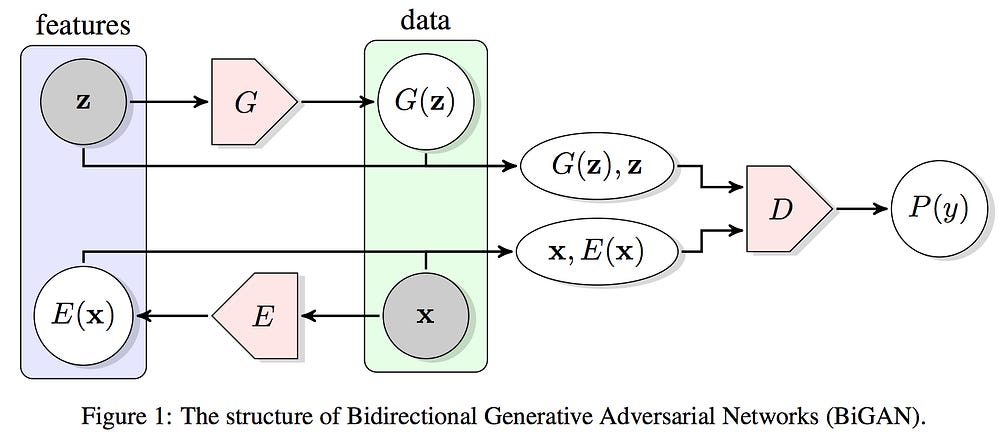Figure source: Donahue et al, 2016 Adversarial Feature Learning

### 2. Interpolation with generative models

With some knowledge of the some of the deep generative models, we’ll examine their capabilities. Generative models are able to learn lower dimensional probability distribution for samples from different classes. Such probability distribution can be used for supervised learning and for generating synthetic samples. While these capabilities are tremendously useful, I am more impressed by generative models’ ability to perform interpolations for real samples along any arbitrary axis to generate non-existent manipulated samples. For example, deep generative models can manipulate images of human faces along axes like age, gender, hair color and etc. In my opinion, this suggests that deep generative models are able to obtain the ability to imagine, as imagination is the process of producing mental images. Next let’s delve into how to perform the interpolation.

The interpolation works by performing simple linear algebra in the latent space (z) learned by the generative model. First, we want to find an axis in the latent space to interpolate along with, which can be something like biological sex. The interpolation vector for biological sex can then be simply computed as the vector pointing from the centroid of males to the centroid of females in the latent space.

More generically, we first need to find the centroids of two classes (a, b) in the latent space:

The interpolation vector in the latent space pointing from class b to class a is:

Given any unseen sample of any class x_c, we can manipulate the unseen sample with the interpolation vector by: 1) encode the sample into the latent space; 2) perform linear interpolation in the latent space; and 3) decode the interpolated sample back to the original space:

α in the above equation is a scalar determining the magnitude and direction of the interpolation. Next, I will play with around the α to slide along different interpolation vectors. The following Python function can make a trained generative model perform such interpolation:

### 3. Experiments of generative models with MNIST data

I trained some generative models, including Naive Bayes, VAE and BiGAN, on the MNIST handwritten digit dataset to experiment with interpolation. Below is a figure visualizing the latent space of a VAE with only two neurons at the bottleneck layer. Although there is some distinctive patterns for the different digits, the reconstruction quality is pretty bad. Perhaps it is challenging to compress 784-dimensional space to 2-d space. I found VAE with 20 neurons at the bottleneck layer can reconstruct the MNIST data with decent quality.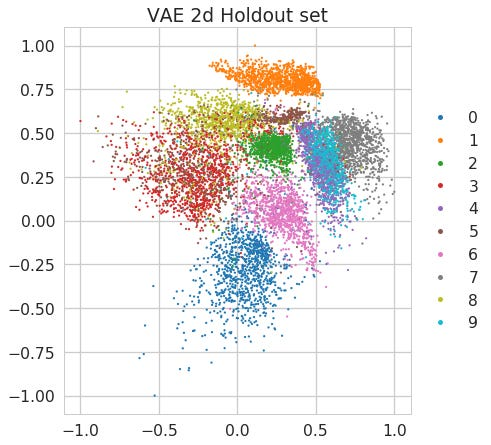Latent space learned by a VAE with 2 neurons at the bottleneck layer

It is also worth pointing out the generative models are trained unsupervisedly. Therefore, the learned latent space has no knowledge of the class labels. The interpolation vectors are calculated after models have finished learning.

To play with the interpolation, I first visualized the interpolation vectors between all the 45 possible pairs of the 10 digits: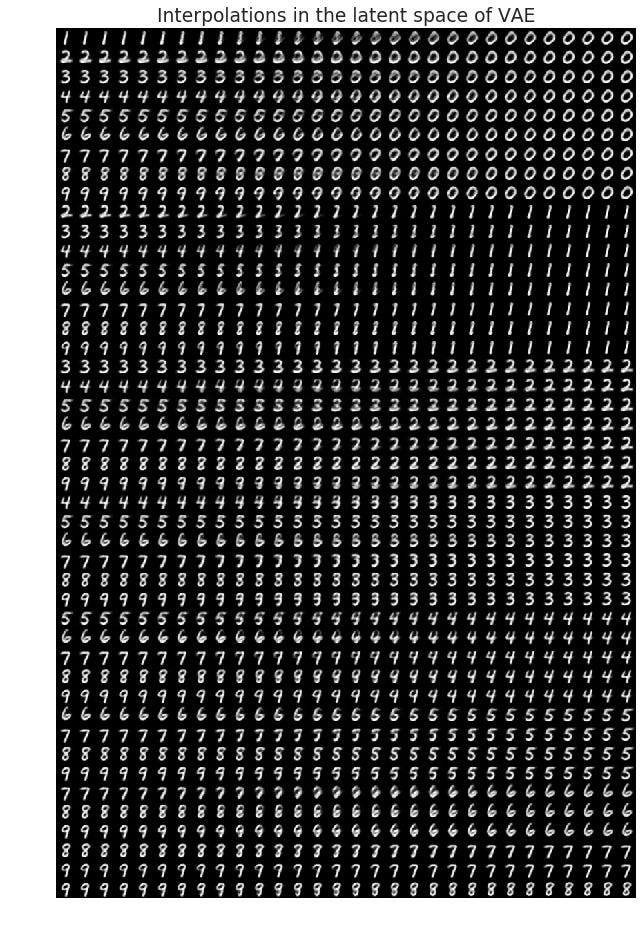Visualization of the interpolation vectors of MNIST digits in the latent space of a VAE with 20 neurons at the bottleneck layer

In the figure above, each row corresponds to a interpolation vector pointing from one digit to another whereas each column corresponds to an alpha value. It is intriguing to look at the digits generated from the latent space from left to right to see how one number gradually change to another. From this we can also find the ambiguous digits that lie between two centroids of our 10 digits.

Next, I did another interesting experiment with the interpolation: I asked whether we can turn a digit 7 to a digit 6 or 0 by moving it along the 6->0 vector. Here are the results of the generated images. It shows some relatively 0 looking images to the right while the left ones do not look like 6 at all.

These images can also be quantified using a Logistic Regression classifier trained on MNIST to predict the probabilities of the labels. And the classifier pretty much agrees with our perception from eyeballing the images.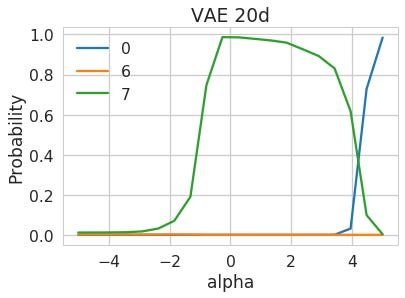Predicted probability for the images of interpolated digits from a Logit classifier

The seemingly boring proof-of-concept experiments with MNIST dataset demonstrated deep generative models’ ability to imagine. I can envision many practical applications with the interpolation.

This post is based on my GitHub repo if you want to get more technical details:

Notebook version of this post presented at Ma’ayan lab meeting: